Wortal Stefana Banacha

# Wortal Stefana Banacha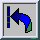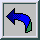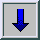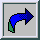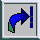"Theory of the Integral"
by Stanisław Saks
with additional notes by Stefan Banach
"Monografie Matematyczne" series, vol. 7, Polish Mathematical Society, Warszawa-Lwów, 1937, p. VII+347.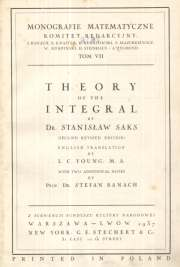COVER PAGE [PDF]
CONTENTS [PDF]
PREFACE [PDF]
ERRATA [PDF]
CHAPTER I. [PDF]
The integral in an abstract space.
§ 1. Introduction
§ 2. Terminology and notation
§ 3. Abstract space X
§ 4. Additive classes of sets
§ 5. Additive functions of a set
§ 6. The variations of an additive function
§ 7. Measurable functions
§ 8. Elementary operations on measurable functions
§ 9. Measure
§ 10. Integral
§ 11. Fundamental properties of the integral
§ 12. Integration of sequences of functions
§ 13. Absolutely continuous additive functions of a set
§ 14. The Lebesgue decomposition of an additive function
§ 15. Change of measure
CHAPTER II. [PDF]
Caratheodory measure.
§ 1. Preliminary remarks
§ 2. Metrical space
§ 3. Continuous and semi-continuous functions
§ 4. Caratheodory measure
§ 5. The operation (A)
§ 6. Regular sets
§ 7. Borel sets
§ 8. Length of a set
§ 9. Complete space
CHAPTER III. [PDF]
Functions of bounded variation and the Lebesgue-Stieltjes integral.
§ 1. Euclidean spaces
§ 2. Intervals and figures
§ 3. Functions of an interval
§ 4. Functions of an interval that are additive and of bounded variation
§ 5. Lebesgue-Stieltjes integral. Lebesgue integral and measure
§ 6. Measure defined by a non-negative additive function of an interval
§ 7. Theorems of Lusin and Vitali-Caratheodory
§ 8. Theorem of Fubini
§ 9. Fubini's theorem in abstract spaces
§ 10. Geometrical definition of the Lebesgue-Stieltjes integral
§ 11. Translations of sets
§ 12. Absolutely continuous functions of an interval
§ 13. Functions of a real variable
§ 14. Integration by parts
CHAPTER IV. [PDF]
Derivation of additive functions of a set and of an interval.
§ 1. Introduction
§ 2. Derivates of functions of a set and of an interval
§ 3. Vitali's Covering Theorem
§ 4. Theorems on measurability of derivates
§ 5. Lebesgue's Theorem
§ 6. Derivation of the indefinite integral
§ 7. The Lebesgue decomposition
§ 8. Rectifiable curves
§ 9. De la Vallee Poussin's theorem
§ 10. Points of density for a set
§ 11. Ward's theorems on derivation of additive functions of an interval 133
§ 12. A theorem of Hardy-Littlewood
§ 13. Strong derivation of the indefinite integral
§ 14. Symmetrical derivates
§ 15. Derivation in abstract spaces
§ 16. Torus space
CHAPTER V. [PDF]
Area of a surface z=F(x,y).
§ 1. Preliminary remarks
§ 2. Area of a surface
§ 3. The Burkill integral
§ 4. Bounded variation and absolute continuity for functions of two variables 169
§ 5. The expressions of de Geöcze
§ 6. Integrals of the expressions of de Geöcze
§ 8. Tonelli's Theorem
CHAPTER VI. [PDF]
Major and minor functions.
§ 1. Introduction
§ 2. Derivation with respect to normal sequences of nets
§ 3. Major and minor functions
§ 4. Derivation with respect to binary sequences of nets
§ 5. Applications to functions of a complex variable
§ 6. The Perron integral
§ 7. Derivates of functions of a real variable
§ 8. The Perron-Stieltjes integral
CHAPTER VII. [PDF]
Functions of generalized bounded variation.
§ 1. Introduction
§ 2. A theorem of Lusin
§ 3. Approximate limits and derivatives
§ 4. Functions VB and VBG
§ 5. Functions AC and ACG
§ 6. Lusin's condition (N)
§ 7. Functions VB* and VBG*
§ 8. Functions AC* and ACCr*
§ 9. Definitions of Denjoy-Luain
§ 10. Criteria for the classes of functions VBG*, ACG*, VBG and ACG
CHAPTER VIII. [PDF]
Denjoy integrals.
§ 1. Descriptive definition of the Denjoy integrals
§ 2. Integration by parts
§ 3. Theorem of Hake-Alexandroff-Looman
§ 4. General notion of integral
§ 5. Constructive definition of the Denjoy integrals
CHAPTER IX. [PDF]
Derivates of functions of one or two real variables.
§ 1. Some elementary theorems
§ 2. Contingent of a set
§ 3. Fundamental theorems on the contingents of plane sets
§ 4. Denjoy's theorems
§ 5. Relative derivates
§ 6. The Banach conditions (T1) and (T1)
§ 7. Three theorems of Banach
§ 8. Superpositions of absolutely continuous functions
§ 9. The condition (D)
§ 10. A theorem of Denjoy-Khintchine on approximate derivates
§ 11. Approximate partial derivates of functions of two variables
§ 12. Total and approximate differentials
§ 13. Fundamental theorems on the contingent of a set in space
§ 14. Extreme differentials
NOTE I. On Haar's measure by Stefan Banach [PDF]
NOTE II. The Lebesgue integral in abstract spaces by Stefan Banach [PDF]
BIBLIOGRAPHY [PDF]
GENERAL INDEX [PDF]
NOTATIONS [PDF]

### From Preface

[...] This volume contains two Notes by S. Banach. The first of them, on Haar's measure, is the translation (with a few slight modifications) of the note already contained in the French edition of this book. The second, which concerns the integration in abstract spaces, is published here for the first time and completes the considerations of Chapter I. [...]
S. Saks.
Warszawa-Żoliborz, July, 1937.

### Acknowledgements

We deeply thank Prof. dr. hab. med. Alina Filipowicz-Banach and the whole of family of Stefan Banach for their permission to post copies of all the works of Stefan Banach on this website. We thank Prof. dr. hab. Stefan Jackowski, the Head of the Polish Mathematical Society, for the permission to post copies of books coauthored by Stefan Banach and published by the Polish Mathematical Society in the series of "Monografie Matematyczne" on this website.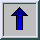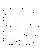Będziemy wdzięczni za wszelkie uwagi i komentarze dotyczące tej strony.